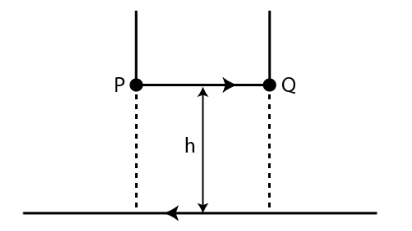# A long straight wire carrying a current of 25 A

Question:

A long straight wire carrying a current of 25 A rests on a table as shown in the figure. Another wire PQ of length 1 m, mass 2.5 g carries the same current but in the opposite direction. The wire PQ is free to slide up and down. To what height will PQ rise?Solution:The magnetic field produced by a long straight current-carrying wire is given as

B = μ0I/2πh

Magnetic force on the small conductor is F = BIl sin θ = BIl

F = mg = μ0I2l/2πh

h = 0.51 cm